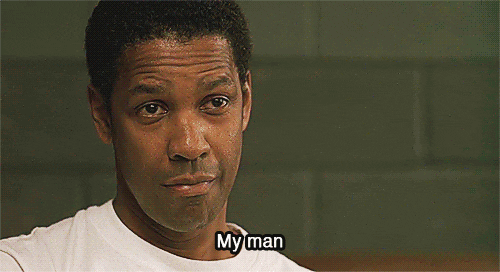# Mental maths

For me, I do:

7 + 9 = 16 (which further breaks down into 9 + 1 = 10, 10 + 6 = 16, but we’ll ignore that)

20 + 80 = 100

100 + 16 = 116

4 Likes

Just have a cry

9 Likes

27+80=107
107+9=116

27+90 = 117
117-1 = 116

3 Likes

20 + 80 = 100
7 + 9 = 16
100 + 16 = 116

14 Likes

89+11=100
27-11=16
100+16=116

3 Likes

Can do maths in my head very well but usually only if it’s prices. Ask me what 287+1562 is and not a clue but £2.87+£15.62? Easy.

I’ve gone cross-eyed trying to do this in my head

2 Likes

Easier to round up the nearest one to a round number then work downwards I find.

200 + 800 = 1000
70 + 90 = 160
1000 - 160 = 840
Remove the zero = 84

# 84

10 Likes2 Likes

7 + 89 = 96
96 + 20 = 116

7 Likes

I like to do the little bits first, not sure why

90+27-1

3 Likes

20+80 then 7+9

1 Like

Don’t engage the brain for less than a grand

3 Likes2 Likes

This, was starting to think I was on my own

2 Likes